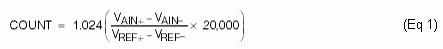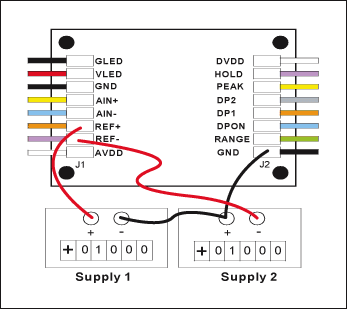# Implementing an External Reference and the Resulting Effect on Gain for the MAX149x Sigma-Delta Panel-Meter ADCs

### Abstract

The MAX149x family of sigma-delta panel-meter ADCs can use an external reference over a 500mV to 3.6V range and achieve accurate conversion results. This application note illustrates how to use an external voltage reference and divide down the output to create a variable reference voltage that drives the MAX149x family and adjusts the ADC's gain.

### How the Reference Voltage Affects the ADC

The MAX1447, MAX1491, MAX1493, MAX1495, MAX1496, MAX1498 sigma-delta panel-meter ICs give the user a choice of either a 2.048V internal or an external reference source between 500mV and 3.6V. In the most basic configuration, these devices expect a 2.048V reference at the input across AIN+ and AIN-, and convert the result to show on the LED/LCD display. The output count is valid for the 4.5-digit version and is given by the following transfer functions. For the 3.5-digit versions, change the 20,000 in these equations to 2000.

With AIN+ - AIN- > 0, RANGE = GNDWith AIN+ - AIN- < 0, RANGE = GNDWith AIN+ - AIN- > 0, RANGE = DVDDWith AIN+ - AIN- < 0, RANGE = DVDD### Building a Simple Adjustable Reference Using a Voltage Divider

Changing the reference voltage changes the gain of the device. Certain applications take advantage of this and use a variable external-reference source to provide adjustable gain.

By adjusting the differential reference voltage term (VREF+ - VREF-) in the numerator of equation 1, it is easy to see the gain change in the transfer function. To increase gain, simply lower the reference voltage. Conversely, to reduce gain, raise the reference voltage. While there are several methods of creating an adjustable reference, the simplest method uses an adjustable voltage divider with a 2.5V reference to vary VAIN+.Figure 1. MAX6126 + divider with REF+ = VOUT and REF- = GND. The expected range of VOUT = 1.5V to 2.5V.

The adjustable reference circuit shown in Figure 1 consists of a 2.5V reference (MAX6126) with a variable resistor in series with a fixed resistor connected from the reference output (OUTF) to GND. The output of this circuit, REF+, connects to the ADC's REF+ and REF- connects to GND. The differential reference voltage, VREF, is given by:The input impedance to the ADC on REF+ is typically 220MΩ, and therefore does not affect equation 2 as long as (RVAR + R) < 1MΩ.

### Practical Issues When Using an External Reference for the MAX149x Family

It is important to note that the MAX1447, MAX1491, MAX1493, MAX1495, MAX1496, MAX1498 restrict the reference input pins to an absolute voltage of ±2.2V for accurate conversions. It is therefore important not to exceed 2.2V on REF+ when using the circuit in Figure 1. In addition, resistors drift with temperature, which can change the ratio of the resistor divider with temperature. Use low-tempco resistors, such as thin-film chip resistors, and avoid carbon resistors and potentiometers. If a fixed gain is desired, it is possible to achieve ±1ppm/°C ratio drift using precision-matched resistor networks such as the MAX5490. (See Figure 2.)Figure 2. Using the MAX5490 precision voltage dividers with the MAX1447, MAX1491, MAX1493, MAX1495, MAX1496, MAX1498 ADCs.

As REF+ and REF- should not exceed ±2.2V, it is necessary to center the external reference voltage around GND when using a reference voltage higher than 2.2V. To achieve this, Figure 3 shows how two external reference sources can be used to generate a voltage for REF- and REF+. The external reference can go as low as 0.5V and as high as 3.6V and still maintain performance. Figure 4 shows the accuracy of a typical device with a 500mV input and a reference voltage between 500mV and 4.2V.Figure 3. Illustration of the MAX1498/MAX1499/MAX1477-based panel meter generating REF+ and REF- using two reference sources.Figure 4. Relative error vs. reference voltage for the circuit in Figure 3.